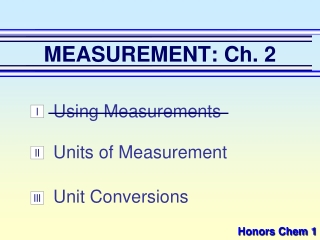DownloadDownload PresentationMEASUREMENT: Ch. 2

# MEASUREMENT: Ch. 2

Download Presentation## MEASUREMENT: Ch. 2

- - - - - - - - - - - - - - - - - - - - - - - - - - - E N D - - - - - - - - - - - - - - - - - - - - - - - - - - -
##### Presentation Transcript

1. MEASUREMENT: Ch. 2 Using Measurements I Units of Measurement II Unit Conversions III Honors Chem 1

2. MEASUREMENT: Ch. 2 Units of Measurement (p.33 - 39)

3. A. Number vs. Quantity Measurements represent quantitiesQuantity is a number + unit UNITS MATTER!!

4. B. SI Units • scientists use one common measurement system • Le Systéme International d’Unités, or SI • Allows scientists to compare their research findings all over the world!

5. B. SI Units Quantity Symbol Base Unit Abbrev. Length l meter m Mass m gram g Time t second s Temp T kelvin K Amount n mole mol

6. B. SI Units K H D b d c m _ _  _ _ n _ _ p kilo deci nano pico centi micro milli base unit

7. Board & Buddy • Shoulder partner • One person grab a white board & marker • Write which unit would be best for the following situations- talk it out!

8. Which unit would be best? distance between Nashville and Orlando

9. Which unit would be best? animal track size

10. Which unit would be best? how long to complete the Nashville Rock n Roll Marathon (26.2 miles)

11. Which unit would be best? size of a paper clip

12. Which unit would be best? how far from street to the top of Big Ben

13. Which unit would be best? amount in a jug of milk

14. Which unit would be best? thickness of a piece of hair

15. Which unit would be best? thickness of a single piece of newspaper

16. Which unit would be best? teaspoon of salt

17. Which unit would be best? distance to the moon

18. m V D = C. Derived Units • combination of base units • volume (m3 or cm3) length  length  length 1 cm3 = 1 mL 1 dm3 = 1 L • density (g/mL or g/cm3)mass per volume

19. D. Density directly proportional! Mass (g) Volume (cm3)

20. m m m m D D D D V V V V D. Density D = m V m = DV V = m D

21. Example #1 • An object has a volume of 825 cm3 and a density of 13.6 g/cm3. Find its mass. m = DV m = (13.6 g/cm3)(825cm3) m = 11,200 g m = 1.12 x 104 g

22. WORK: V = m D V = 25 g 0.87 g/mL Example #2 • A liquid has a density of 0.87 g/mL. What volume is occupied by 25 g of the liquid? V = 29 mL

23. E. Converting temperatures • Celcius (°C) • Kelvin (K) • Fahrenheit (°F) K = °C + 273 °C = K - 273